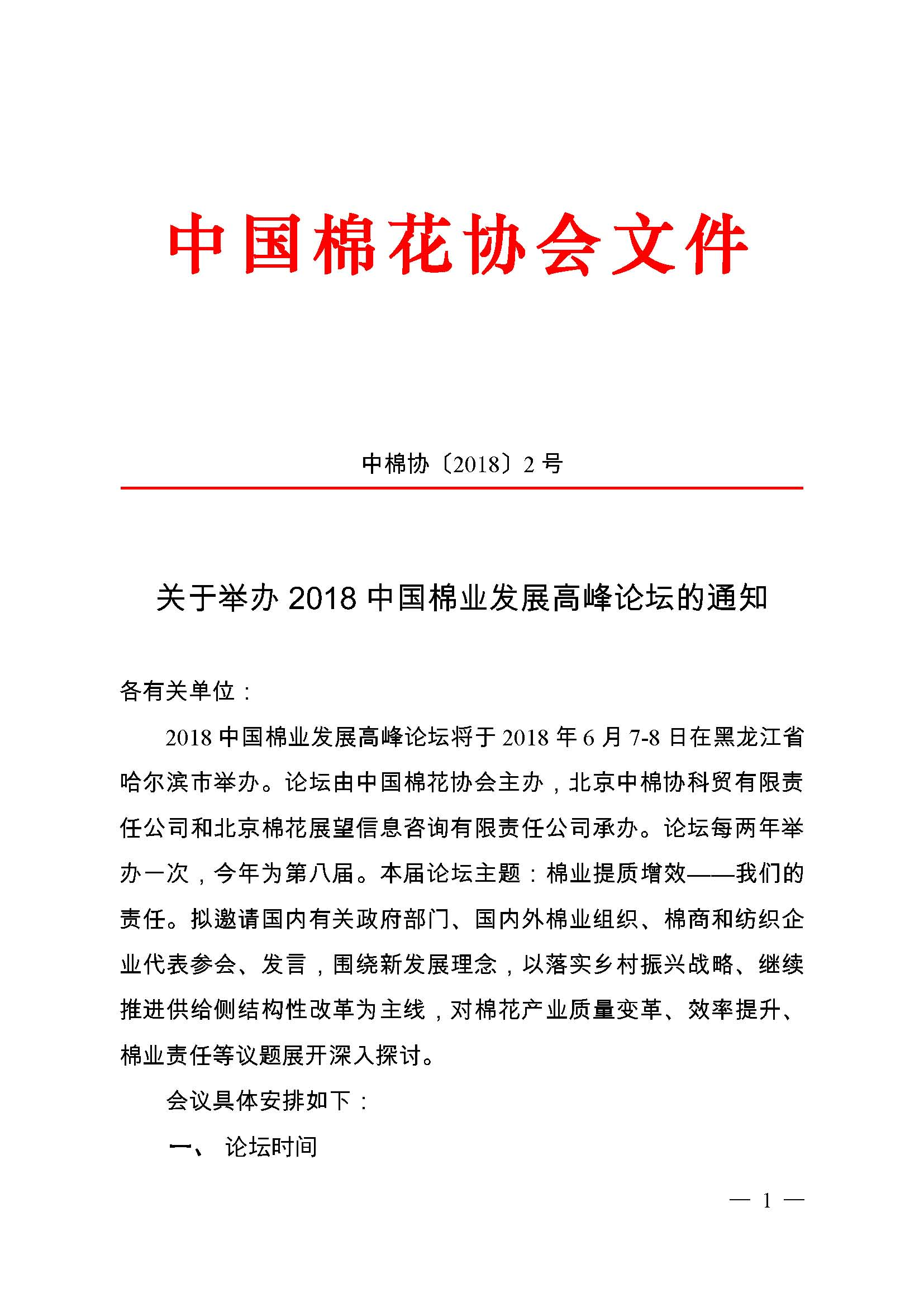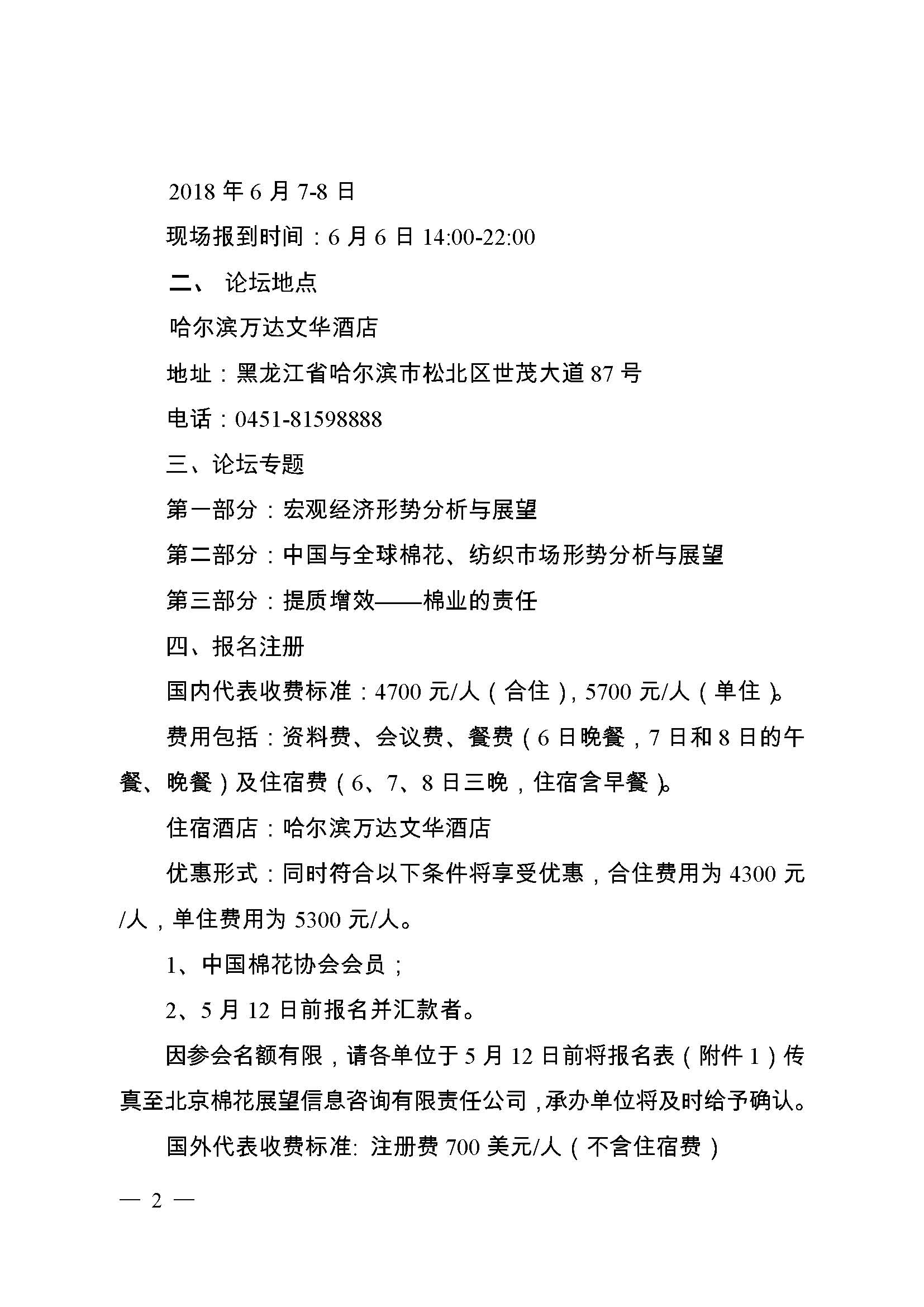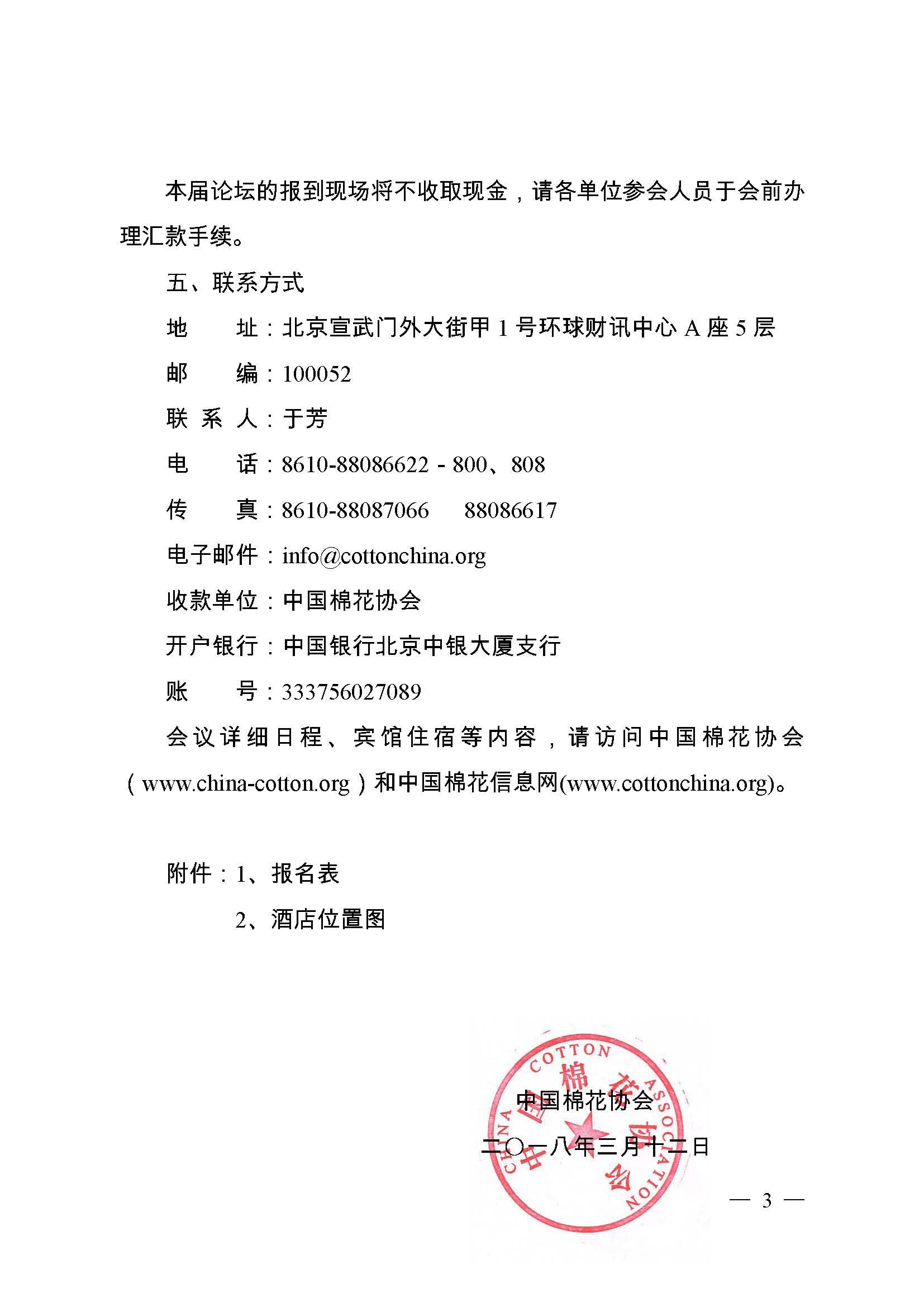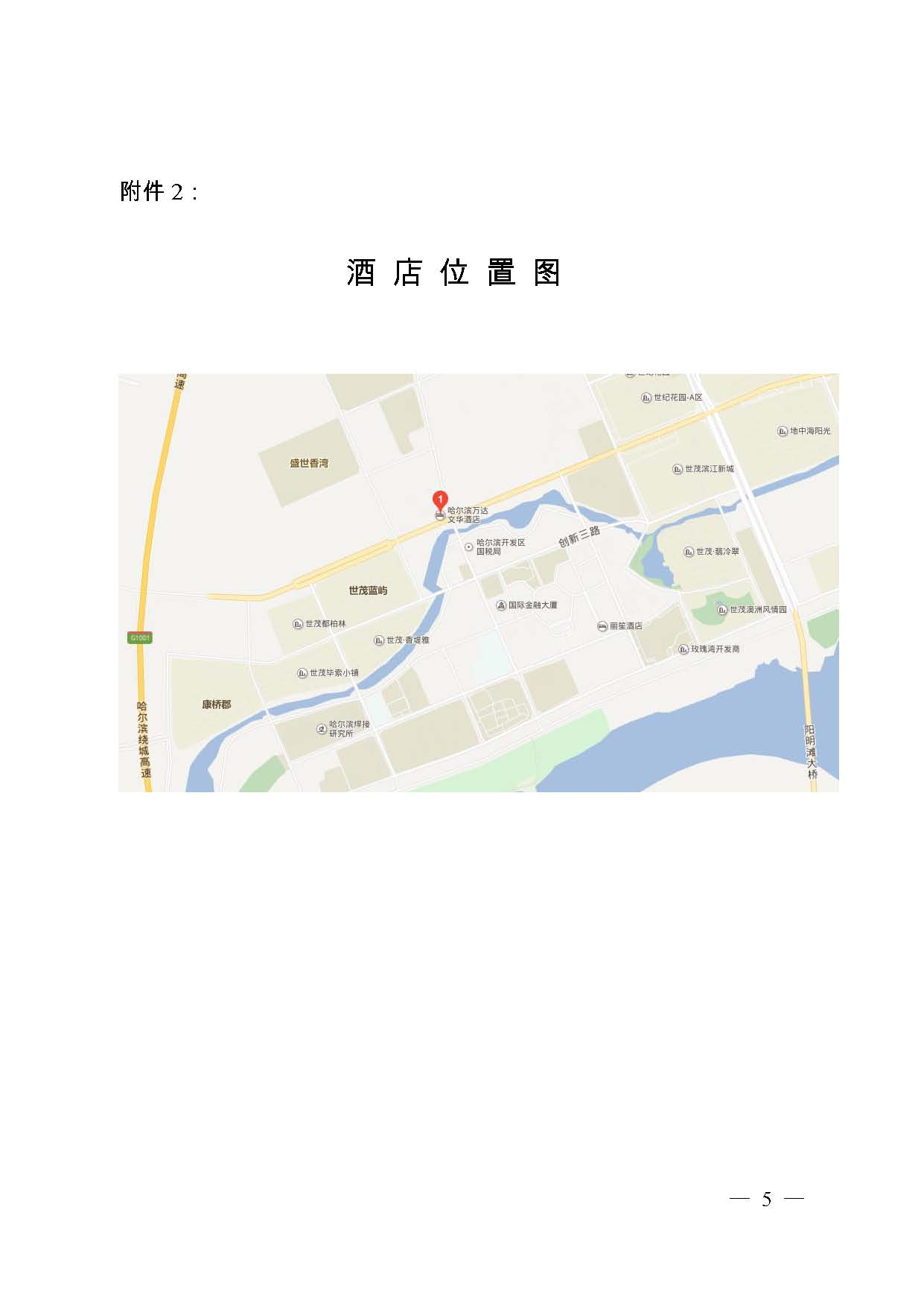# 关于举办 2018中国棉业发展高峰论坛的通知```

```
`	`

```

```
`	`

```

```
`	`

```

```
`	`

```

```
`	`

```

```
`	`

```

```
`	`

```

```
`	`

```

```
`	`

```

```
`	`

```

```
`	`

```

```
`	`

```

```
`	`

```

```
`	`

```

```
`	`

```

```
`	`

```

```
`	`

```

```
`	`

```

```
`	`

```

```
`	`

```

```
`	`

```

```
`	`

```

```
`	`

```

```
`	`

```

```
`	`

```

```
`	`

```

```
`	`

```

```
`	`

```

```
`	`

```

```
`	`

```

```
`	`

```

```
`	`

```

```
`	`

```

```
`	`

```

```
`	`

```

```
`	`

```

```
`	`

```

```
`	`

```

```
`	`

```

```
`	`

```

```
`	`

```

```
`	`

```

```
`	`

```

```
`	`

```

```
`	`

```

```
`	`

```

```
`	`

```

```
`	`

```

```
`	`

```

```
`	`

```

```
`	`

```

```
`	`

```

```
`	`

```

```
`	`

```

```
`	`

```

```
`	`

```

```
`	`

```

```
`	`

```

```
`	`

```

```
`	`

```

```
`	`

```

```
`	`

```

```
`	`

```

```
`	`

```

```
`	`

```

```
`	`

```

```
`	`

```

```
`	`

```

```
`	`

```

```
`	`

```

```
`	`

```

```
`	`

```

```
`	`

```

```
`	`

```

```
`	`

```

```
`	`

```

```
`	`

```

```
`	`

```

```
`	`

```

```
`	`

```

```
`	`

```

```
`	`

```

```
`	`

```

```
`	`

```

```
`	`

```

```
`	`

```

```
`	`

```

```
`	`

```

```
`	`

```

```
`	`

```

```
`	`

```

```
`	`

```

```
`	`

```

```
`	`

```

```
`	`

```

```
`	`

```

```
`	`

```

```
`	`

```

```
`	`

```

```
`	`

```

```
`	`

```

```
`	`

```

```
`	`

```

```
`	`

```

```
`	`

```

```
`	`

```

```
`	`

```

```
`	`

```

```
`	`

```

```
`	`

```

```
`	`

```

```
`	`

```

```
`	`

```

```
`	`

```

```
`	`

```

```
`	`

```

```
`	`

```

```
`	`